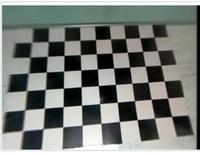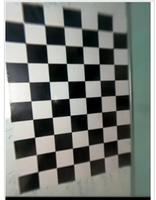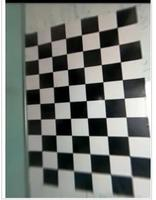# Camera Calibration with Python – OpenCV

• Last Updated : 09 Jun, 2021

Prerequisites: OpenCV

A camera is an integral part of several domains like robotics, space exploration, etc camera is playing a major role. It helps to capture each and every moment and helpful for many analyses. In order to use the camera as a visual sensor, we should know the parameters of the camera. Camera Calibration is nothing but estimating the parameters of a camera, parameters about the camera are required to determine an accurate relationship between a 3D point in the real world and its corresponding 2D projection (pixel) in the image captured by that calibrated camera.

Attention geek! Strengthen your foundations with the Python Programming Foundation Course and learn the basics.

To begin with, your interview preparations Enhance your Data Structures concepts with the Python DS Course. And to begin with your Machine Learning Journey, join the Machine Learning - Basic Level Course

We need to consider both internal parameters like focal length, optical center, and radial distortion coefficients of the lens etc., and external parameters like rotation and translation of the camera with respect to some real world coordinate system.

### Required libraries:

• OpenCV library in python is a computer vision library, mostly used for image processing, video processing, and analysis, facial recognition and detection, etc.
• Numpy is a general-purpose array-processing package. It provides a high-performance multidimensional array object and tools for working with these arrays.

### Camera Calibration can be done in a step-by-step approach:

• Step 1: First define real world coordinates of 3D points using known size of checkerboard pattern.
• Step 2: Different viewpoints of check-board image is captured.
• Step 3: findChessboardCorners() is a method in OpenCV and used to find pixel coordinates (u, v) for each 3D point in different images
• Step 4: Then calibrateCamera() method is used to find camera parameters.

It will take our calculated (threedpoints, twodpoints, grayColor.shape[::-1], None, None) as parameters and returns list having elements as Camera matrix, Distortion coefficient, Rotation Vectors, and Translation Vectors.

Camera Matrix helps to transform 3D objects points to 2D image points and the Distortion Coefficient returns the position of the camera in the world, with the values of Rotation and Translation vectors

Below is the complete program of the above approach:

## Python3

 `# Import required modules``import` `cv2``import` `numpy as np``import` `os``import` `glob`  `# Define the dimensions of checkerboard``CHECKERBOARD ``=` `(``6``, ``9``)`  `# stop the iteration when specified``# accuracy, epsilon, is reached or``# specified number of iterations are completed.``criteria ``=` `(cv2.TERM_CRITERIA_EPS ``+``            ``cv2.TERM_CRITERIA_MAX_ITER, ``30``, ``0.001``)`  `# Vector for 3D points``threedpoints ``=` `[]` `# Vector for 2D points``twodpoints ``=` `[]`  `#  3D points real world coordinates``objectp3d ``=` `np.zeros((``1``, CHECKERBOARD[``0``]``                      ``*` `CHECKERBOARD[``1``],``                      ``3``), np.float32)``objectp3d[``0``, :, :``2``] ``=` `np.mgrid[``0``:CHECKERBOARD[``0``],``                               ``0``:CHECKERBOARD[``1``]].T.reshape(``-``1``, ``2``)``prev_img_shape ``=` `None`  `# Extracting path of individual image stored``# in a given directory. Since no path is``# specified, it will take current directory``# jpg files alone``images ``=` `glob.glob(``'*.jpg'``)` `for` `filename ``in` `images:``    ``image ``=` `cv2.imread(filename)``    ``grayColor ``=` `cv2.cvtColor(image, cv2.COLOR_BGR2GRAY)` `    ``# Find the chess board corners``    ``# If desired number of corners are``    ``# found in the image then ret = true``    ``ret, corners ``=` `cv2.findChessboardCorners(``                    ``grayColor, CHECKERBOARD,``                    ``cv2.CALIB_CB_ADAPTIVE_THRESH``                    ``+` `cv2.CALIB_CB_FAST_CHECK ``+``                    ``cv2.CALIB_CB_NORMALIZE_IMAGE)` `    ``# If desired number of corners can be detected then,``    ``# refine the pixel coordinates and display``    ``# them on the images of checker board``    ``if` `ret ``=``=` `True``:``        ``threedpoints.append(objectp3d)` `        ``# Refining pixel coordinates``        ``# for given 2d points.``        ``corners2 ``=` `cv2.cornerSubPix(``            ``grayColor, corners, (``11``, ``11``), (``-``1``, ``-``1``), criteria)` `        ``twodpoints.append(corners2)` `        ``# Draw and display the corners``        ``image ``=` `cv2.drawChessboardCorners(image,``                                          ``CHECKERBOARD,``                                          ``corners2, ret)` `    ``cv2.imshow(``'img'``, image)``    ``cv2.waitKey(``0``)` `cv2.destroyAllWindows()` `h, w ``=` `image.shape[:``2``]`  `# Perform camera calibration by``# passing the value of above found out 3D points (threedpoints)``# and its corresponding pixel coordinates of the``# detected corners (twodpoints)``ret, matrix, distortion, r_vecs, t_vecs ``=` `cv2.calibrateCamera(``    ``threedpoints, twodpoints, grayColor.shape[::``-``1``], ``None``, ``None``)`  `# Displaying required output``print``(``" Camera matrix:"``)``print``(matrix)` `print``(``"\n Distortion coefficient:"``)``print``(distortion)` `print``(``"\n Rotation Vectors:"``)``print``(r_vecs)` `print``(``"\n Translation Vectors:"``)``print``(t_vecs)`

Input:Output:

``` Camera matrix:
[[ 36.26378216   0.         125.68539168]
[  0.          36.76607372 142.49821147]
[  0.           0.           1.        ]]

Distortion coefficient:
[[-1.25491812e-03  9.89269357e-05 -2.89077718e-03  4.52760939e-04
-3.29964245e-06]]

Rotation Vectors:
[array([[-0.05767492],
[ 0.03549497],
[ 1.50906953]]), array([[-0.09301982],
[-0.01034321],
[ 3.07733805]]), array([[-0.02175332],
[ 0.05611105],
[-0.07308161]])]

Translation Vectors:
[array([[ 4.63047351],
[-3.74281386],
[ 1.64238108]]), array([[2.31648737],
[3.98801521],
[1.64584622]]), array([[-3.17548808],
[-3.46022466],
[ 1.68200157]])]```

My Personal Notes arrow_drop_up